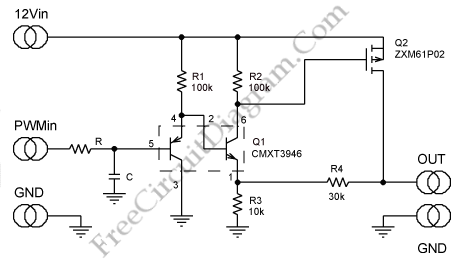# PWM to Amplified and Buffered Linear Signal ConverterThis is a simple circuit that can be used to convert a low-voltage PWM signal into  a buffered and amplified linear output. This circuit is designed for fan speed control. It uses PWM outputs to control fan speed as a function of temperature. Here is the circuit:As a linear amplifier, this circuit uses a PMOS FET (Q2) and a pair of BJTs (Q1). The emitter of the NPN is the inverting input and the non-inverting input to the amplifier is the base of the PNP in Q1. As an emitter follower and as the initial gain element this circuit uses the NPN and the PNP is biased as an emitter follower. The two input voltages approximately track each other because the NPN and the PNP operate at roughly the same  temperature and current density. The amplifier output is the VGS of Q2 that is amplified at Q2’s drain because of the drop across R2. The output offset of the amplifier primarily is about 100mV because the drop across R3 forces the VGS threshold of Q2.  To provide linear control of a 12V fan, this circuit allows a 3.3V input.

To reduce the PWM ripple at the output we must select a cutoff frequency, 1 / [6.28 * R * C] at least two decades below the PWM. The high input impedance of the circuit led to the use of A resistor value as high as 100k. [Source: maxim-ic.com]